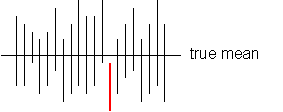## Frequentists and Bayesians

#### Confidence Intervals vs Credible Intervals

Most engineers are surprised to learn that statistics is not monolithic, nor statisticians of one stripe. In fact statistics as a discipline remains sharply divided even on the fundamental definition of “probability.”

The frequentist definition sees probability as the long-run expected frequency of occurrence. $$P(A) = n/N$$, where $$n$$ is the number of times event $$A$$ occurs in $$N$$ opportunities. The Bayesian view of probability is related to degree of belief. It is a measure of the plausibility of an event given incomplete knowledge.

Thus a frequentist believes that a population mean is real, but unknown, and unknowable, and can only be estimated from the data. Knowing the distribution for the sample mean, he constructs a confidence interval, centered at the sample mean.

Here it gets tricky. Either the true mean is in the interval or it is not. So the frequentist can’t say there’s a 95% probability$$^1$$ that the true mean is in this interval, because it’s either already in, or it’s not. And that’s because to a frequentist the true mean, being a single fixed value, doesn’t have a distribution . The sample mean does. Thus the frequentist must use circumlocutions like “95% of similar intervals would contain the true mean, if each interval were constructed from a different random sample like this one.”

Graphically this is illustrated here:Bayesians have an altogether different world-view. They say that only the data are real. The population mean is an abstraction, and as such some values are more believable than others based on the data and their prior beliefs. (Sometimes the prior belief is very non-informative, however.) The Bayesian constructs a credible interval, centered near the sample mean, but tempered by “prior” beliefs concerning the mean.

Now the Bayesian can say what the Frequentist cannot: “There is a 95% probability$$^2$$ that this interval contains the mean.”

##### Notes:
1. probability” = long-run fraction having this characteristic.
2. probability” = degree of believability.
3. A Frequentist is a person whose long-run ambition is to be wrong 5% of the time.
4. A Bayesian is one who, vaguely expecting a horse, and catching a glimpse of a donkey, strongly believes he has seen a mule.